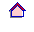行政院農業委員會台南區農業改良場  研究彙報第14號

Fertilization and Potential Yield of Various Up-land Crops in Chia-nan Area

Y. H. Cheng, H. Y. Huang

Summary

To test the potential yield of corn (Shynejea, Matou), sorghum (Putzu), peanut (Tungshyh) and soybean (Yitzu) for understanding the difference of potential yield under culturing and natural condition, the study had been done at Shynejea (10), Matou (10), Yitzu (5) and Tungshyh(5) where fertilization had been demonstrated in 1979.  Three treatments as follows: 1. Demonstration plot-recommending the quantity of fertilizer depended on the soil analysis.  2. Without phosphate plot- the same as treatment 1.  Without phosphate.  3. Check plot – following the usual fertilizer by farmer.

We got the result as the yield of demonstration plots was the highest, and that without phosphate plots was the lowest.  The quantity of phosphate at check plot was less than that at demonstration plot but the quantity of nitrogen was the same at both plots.  Because the cultural technique has raised to the level so the potential yield is small at Shynejea, Matou and Putzu, and if using the correct fertilization the potential yield is larger at Putzu and Tungshyh.回研究彙報目錄